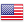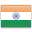Grade "A+" Accredited by NAAC with a CGPA of 3.46
Grade "A+" Accredited by NAAC with a CGPA of 3.46

# Design and Analysis of Algorithms

Course ID
BHCS 08
Level
Program
B.Sc. CS (Hons.)
Semester
Fourth
Credits
6.0
Paper Type
Core Course
Method
Lecture & Practical

## Unique Paper Code: Update Awaited

This course is designed to introduce the students to design and analyse algorithms in terms of efficiency and correctness. The course focuses on highlighting difference between various problem solving techniques for efficient algorithm design.

## Learning Outcomes:

At the end of the course, students should be able to:

• Given an algorithm, identify the problem it solves.
• Write algorithms choosing the best one or a combination of two or more of the algorithm design techniques: Iterative, divide-n-conquer, Greedy, Dynamic Programming using appropriate data structures.
• Write proofs for correctness of algorithms.
• Re-write a given algorithm replacing the (algorithm design) technique used with a more appropriate/efficient (algorithm design) technique.

## Course Contents

Unit 1
Unit 2
Unit 3
Unit 4

Unit 1

Algorithm Design Techniques: Iterative technique: Applications to Sorting and Searching (review), their correctness and analysis. Divide and Conquer: Application to Sorting and Searching (review of binary search), merge sort, quick sort, their correctness and analysis.
Dynamic Programming: Application to various problems (for reference; Weighted Interval Scheduling, Sequence Alignment, Knapsack), their correctness and analysis. Greedy Algorithms: Application to various problems, their correctness and analysis.

Unit 2

More on Sorting and Searching: Heapsort, Lower Bounds using decision trees, sorting in Linear Time – Bucket Sort, Radix Sort and Count Sort, Medians & Order Statistics, complexity analysis and their correctness.

Unit 3

Unit 4

Graphs: Graph Algorithms – Breadth First Search, Depth First Search and its Applications.

### Practicals

#### Lab List 1

1. a)Implement Insertion Sort (The program should report the number of comparisons)
2. b)Implement Merge Sort(The program should report the number of comparisons)

3. Implement Heap Sort (The program should report the number of comparisons)
4. Implement Randomized Quick sort (The program should report the number of comparisons)
6. Create a Red-Black Tree and perform following operations on it: i. Insert a node ii. Delete a node iii. Search for a number & also report the color of the node containing this number.
7. Write a program to determine the LCS of two given sequences
8. Implement Breadth-First Search in a graph
9. Implement Depth-First Search in a graph
10. Write a program to determine the minimum spanning tree of a graph
For the algorithms at S.No 1 to 3 test run the algorithm on 100 different inputs of sizes varying from 30 to 1000. Count the number of comparisons and draw the graph. Compare it with a graph of nlogn.

#### Text Books

Kleinberg, J., & Tardos, E. (2013). Algorithm Design. 1st edition. Pearson Education India.

Cormen, T.H., Leiserson,C.E. Rivest, R.L., & Stein, C.(2015). Introduction to Algorithms. 3rd edition. PHI.
Sarabasse & Gleder A. V. (1999). Computer Algorithm – Introduction to Design and Analysis. 3rd edition. Pearson Education.

#### Teaching Learning Process

Use of ICT tools in conjunction with traditional class room teaching methods
Interactive sessions
Class discussions

#### Assessment Methods

Written tests, assignments, quizzes, presentations as announced by the instructor in the class

#### Keywords

Brute Force Algorithm, divide and conquer, greedy, dynamic programming approaches, inplace algorithm, best / average / worst case running time of algorithms.

Disclaimer: Details on this page are subject to change as per University of Delhi guidelines. For latest update in this regard please refer to the University of Delhi website here.Englishहिन्दी